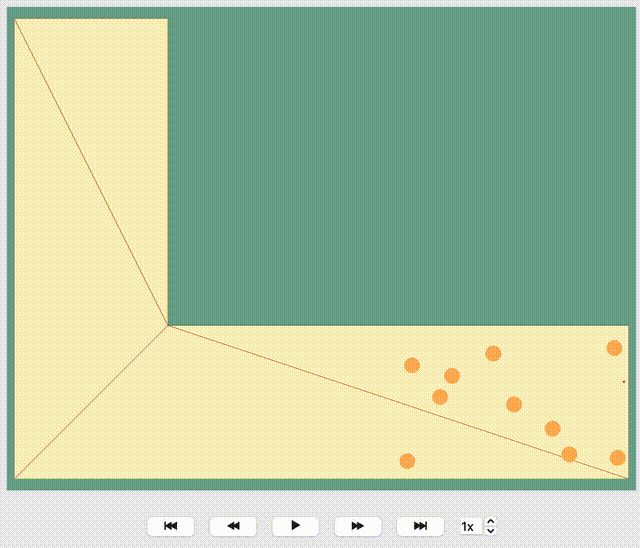# How to work with Journeys#

With JuPedSim, directing agents towards exits and ensuring a smooth evacuation from the simulation area is straightforward and versatile. There might be scenarios where it’s vital to navigate agents along various paths, thus creating diverse evacuation situations. Let’s explore different routing strategies of agents using a simple geometric space - a corner.

JuPedSim manages routing by geometrically triangulating the simulation area. Without user-defined routing strategies, agents, for example, in a corner simulation, naturally move towards the inner edge of the corner. Look at this visualization where the given direction of each agent is shown by a red line. You’ll observe all red lines lead towards the exit along the inner edge of the corner. While it seems logical, this path isn’t always optimal and could result in a bottleneck, thereby slowing down the evacuation process.Hide code cell source
from shapely import Polygon
import pathlib
import pandas as pd
import numpy as np
import jupedsim as jps
import pedpy
from pedpy.column_identifier import ID_COL, FRAME_COL
import matplotlib.pyplot as plt
from matplotlib.patches import Circle

%matplotlib inline


## Preparing the Simulation: Geometry and Routing Instructions#

Let’s start by setting up a basic polygon. This will serve as our main simulation area where agents will be distributed. Additionally, we’ll mark an exit area using another polygon. When agents enter this exit area, they’re deemed to have safely evacuated and will be removed from the ongoing simulation.

Next, we’ll introduce an initial target for the agents: a sizable circular area (known as a switch). After the simulation kickstarts, agents will first head towards this circle. Once they enter the circle, they’ll be directed to one of three distinct waypoints, set diagonally along the curve of the corner.

For the simulation’s onset, all agents will be positioned inside a rectangular zone at the corner’s base.

simulation_polygon = Polygon(
[(-7, 15), (-7, -7), (23, -7), (23, 0), (0, 0), (0, 15)]
)
exit_polygon = [(-6.8, 14.8), (-0.2, 14.8), (-0.2, 13.5), (-6.8, 13.5)]
switch_point = (7, -3.5)
waypoints = [
(-0.5, -0.5),
(-3, -2),
(-6, -4),
]
distance_to_waypoints = 3
distance_to_switch = 3

distribution_polygon = Polygon(
[[22.8, -0.3], [10.8, -0.3], [10.8, -6.8], [22.8, -6.8]]
)
walkable_area = pedpy.WalkableArea(simulation_polygon)

Hide code cell source
fig, ax = plt.subplots(nrows=1, ncols=1)
ax.set_aspect("equal")
pedpy.plot_walkable_area(walkable_area=walkable_area, axes=ax)

x, y = distribution_polygon.exterior.xy
plt.fill(x, y, alpha=0.1)
plt.plot(x, y, color="white")
centroid = distribution_polygon.centroid
plt.text(
centroid.x, centroid.y, "Start", ha="center", va="center", fontsize=10
)

x, y = Polygon(exit_polygon).exterior.xy
plt.fill(x, y, alpha=0.1)
plt.plot(x, y, color="white")
centroid = Polygon(exit_polygon).centroid
plt.text(centroid.x, centroid.y, "Exit", ha="center", va="center", fontsize=10)

ax.plot(switch_point, switch_point, "bo")
circle = Circle(
(switch_point, switch_point),
distance_to_switch,
fc="blue",
ec="blue",
alpha=0.1,
)
ax.annotate(
f"Switch",
(switch_point, switch_point),
textcoords="offset points",
xytext=(-5, -15),
ha="center",
)
for idx, waypoint in enumerate(waypoints):
ax.plot(waypoint, waypoint, "ro")
ax.annotate(
f"WP {idx+1}",
(waypoint, waypoint),
textcoords="offset points",
xytext=(10, -15),
ha="center",
)
circle = Circle(
(waypoint, waypoint),
distance_to_waypoints,
fc="red",
ec="red",
alpha=0.1,
)num_agents = 100
positions = jps.distribute_by_number(
polygon=distribution_polygon,
number_of_agents=num_agents,
distance_to_agents=0.4,
seed=12,
distance_to_polygon=0.2,
)


## Exploring Transition Strategies#

All agents initially set their course towards the switch_point. After reaching it, they navigate towards intermediate goals (waypoints) before making their way to the final exit. The challenge lies in deciding which waypoint each agent should target next.

Let’s explore three unique methods to determine these transition strategies:

1. Direct Path Strategy: Here, every agent simply aims for the first waypoint, mirroring a shortest path algorithm.

2. Balanced Load Strategy: Agents are directed towards the least occupied waypoint, ensuring a more balanced distribution.

3. Round Robin Strategy: Waypoints are sequentially assigned to agents, rotating through each in turn.

### Direct Path Strategy#

def shortest_path(
simulation: jps.Simulation, switch_id, waypoint_ids, exit_id
):
"""Build a journey with fixed transitions for a given simulation."""

journey = jps.JourneyDescription([switch_id, *waypoint_ids, exit_id])
# switch ---> 1st waypoint
journey.set_transition_for_stage(
switch_id, jps.Transition.create_fixed_transition(waypoint_ids)
)
# 1st waypoint ---> exit
journey.set_transition_for_stage(
waypoint_ids, jps.Transition.create_fixed_transition(exit_id)
)

return journey_id


def least_targeted(
simulation: jps.Simulation, switch_id, waypoint_ids, exit_id
):
"""Build a journey with least targeted transitions for a given simulation."""

journey = jps.JourneyDescription([switch_id, *waypoint_ids, exit_id])
# switch ---> least targeted waypoint
journey.set_transition_for_stage(
switch_id,
jps.Transition.create_least_targeted_transition(waypoint_ids),
)
# from all waypoints ---> exit
for waypoint_id in waypoint_ids:
journey.set_transition_for_stage(
waypoint_id, jps.Transition.create_fixed_transition(exit_id)
)

return journey_id


### Round Robin Strategy#

def round_robin(simulation: jps.Simulation, switch_id, waypoint_ids, exit_id):
"""Build a journey with least round-robin transitions for a given simulation."""

journey = jps.JourneyDescription([switch_id, *waypoint_ids, exit_id])
# switch ---> 1st waypoint with weight1
# switch ---> 2nd waypoint with weight2
# switch ---> 3th waypoint with weight3
weight1, weight2, weight3 = 1, 1, 1
journey.set_transition_for_stage(
switch_id,
jps.Transition.create_round_robin_transition(
[
(waypoint_ids, weight1),
(waypoint_ids, weight2),
(waypoint_ids, weight3),
]
),
)
# from all waypoints ---> exit
for waypoint_id in waypoint_ids:
journey.set_transition_for_stage(
waypoint_id, jps.Transition.create_fixed_transition(exit_id)
)

return journey_id

scenarios = [
shortest_path,
least_targeted,
round_robin,
]


## Executing the Simulation#

With all components in place, we’re set to initiate the simulation. For this demonstration, the trajectories will be recorded in an sqlite database.

First we setup some agent parameters then run three simulation with the different strategies:

def run_scenario_simulation(scenario, agent_parameters, positions, geometry):
"""Runs a simulation for a given scenario using the provided simulation object, agent parameters, and positions."""
filename = f"{scenario.__name__}.sqlite"

simulation = jps.Simulation(
dt=0.05,
model=jps.CollisionFreeSpeedModel(
strength_neighbor_repulsion=2.6,
range_neighbor_repulsion=0.1,
range_geometry_repulsion=0.05,
),
geometry=geometry,
trajectory_writer=jps.SqliteTrajectoryWriter(
output_file=pathlib.Path(filename)
),
)
waypoint_ids = [
for waypoint in waypoints
]
agent_parameters.stage_id = switch_id
journey_id = scenario(simulation, switch_id, waypoint_ids, exit_id)
agent_parameters.journey_id = journey_id
for new_pos in positions:
agent_parameters.position = new_pos

while simulation.agent_count() > 0:
simulation.iterate()

return filename, simulation.iteration_count()

for scenario in scenarios:
filename, iteration_count = run_scenario_simulation(
scenario,
jps.CollisionFreeSpeedModelAgentParameters(),
positions,
walkable_area.polygon,
)


## Visualizing the Trajectories#

To visualize trajectories, we’ll pull simulation data from the SQLite database and then employ a helper function to depict the agent movements. For subsequent analyses, we’ll organize these trajectory files within a dictionary for easy access.

from jupedsim.internal.notebook_utils import animate, read_sqlite_file

agent_trajectories = {}
for scenario in scenarios:
scenario_name = scenario.__name__